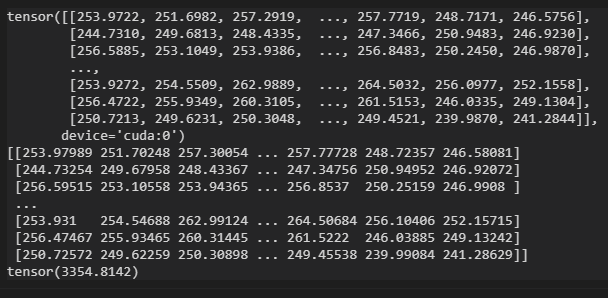# Is there a big problem with pytoch's matrix multiplication?

I compared the pytorch’s matrix multiplication with numpy’s, and discovered that they were very different.
The experimental code is as follow：

``````import torch

a = torch.rand([1000,1000],device='cuda')
b = torch.mm(a,a)
print(b)
a = a.cpu().numpy()
c = a.dot(a)
print(c)
print(torch.sum(torch.abs((b.cpu()-c))))
``````

The output results are as follows:We can see that the absolute error of the result of the multiplication of two matrices reaches 3000.

Hy there, I guess it’s about precision, the error is due to float 32 which causes this round off error.

``````import torch
a = torch.rand([1000,1000],device='cuda',dtype=torch.float64)
torch.set_printoptions(7)
b = torch.mm(a,a)
print(b)
a = a.cpu().numpy()
c = a.dot(a)
print(c)
print(torch.sum(torch.abs((b.cpu()-c))))
``````Thank you! I guess numpy might first transform the inputs to float64 before matrix multiplication.